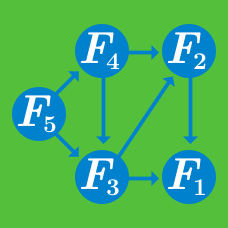Computer Science

Dynamic Programming: Level 5 Challenges

The sequence $s = [ 1, 2, 2, 1, 1, 2, 1, 2, 2, 1, 2, 2, 1, 1, 2, 1, 1, 2, 2, 1, 2, \dots ]$ may be described as

1 one, 2 twos, 2 ones, 1 two, 1 one, 2 twos, 1 one, etc.

Remarkably, the counts that occur in this description are precisely the elements of $s$ itself! Thus, $s$ is a self-describing sequence.

$\underbrace{1}_{1}\ \underbrace{2\ 2}_{2}\ \underbrace{1\ 1}_{2}\ \underbrace{2}_{1}\ \underbrace{1}_{1}\ \underbrace{2\ 2}_{2}\ \underbrace{1}_{1}\ \underbrace{2\ 2}_{2}\ \underbrace{1\ 1}_{2}\ \underbrace{2}_{1}\ \underbrace{1\ 1}_{2}\ \cdots$

How many twos occur in the first 1,000,000 elements of this sequence?

Bonus: In the original version of this problem, no more than 2000 bytes of memory was to be used during the calculation.

Source: A computer programming contest between Dutch students and professionals a long time ago (1996 or 1997 if I remember correctly.) Our team was the only team to get this problem right, and that within the first five minutes of the contest. Can you beat our record? :)

Here is a set $S$ of numbers.

There is some subset $X \subset S$ such that all the elements of $X$ form an arithmetic progression when sorted.

What is the largest possible value of $|X|$?

Extra Credit: Minimize the time complexity of your solution.Consider an image, fully-detailed, perfectly square, but initially represented as but a single, uniform pixel, the average of all of its contents. Naturally, one would wish to see more - more details, more pixels, more depth, but receiving all of this information instantaneously, perfectly, can be so... boring.

Instead, let us explore the image, piece by piece, quadrant by quadrant. The first pixel is given to us for free, and to see more, we must explore it - digging deeper, one 'pixel' may be split into four, each being the average of the top-left, top-right, bottom-left, and bottom-right subspaces it covers respectively, all equal in size. Each of these, we may then, in turn, explore, revealing more and more detail as we go, until the pixels revealed are, in fact, pixels, yielding no further detail than themselves.

In this way, what was once a fixed, linear journey - row by row, column by column - has become something much richer! There are many different ways one could reveal the full landscape, many different paths one could take to reveal each detail. For a $1 \times 1$ image, or a $2 \times 2$ image, there is but one way forward, but for a $4 \times 4$ image, there are $24$ ways to reveal everything! One touch on the center to reveal $4$ distinct $2 \times 2$ 'pixels', which can, of course, be explored in $4!$ different orders to reveal the entire contents of the landscape. However, this number gets much richer, very quickly.

A $8 \times 8$ image can be fully explored in $3892643213082624$ ways. That's right, over $3$ quadrillion paths can be taken.

Let $N$ be the number of ways a $512 \times 512$ landscape can be fully explored. The number $N$ has over three-hundred thousand decimal digits, so calculating it is no mere pencil-and-paper operation.

What is the digit-sum of $N$?

Inspired by this class of interactive animation.

(Took me a while to find a link! Hard to search for, these were somewhat popular a couple years ago.)